• 梯度下降法公式推导 ** 梯度下降法简单的来说就是一种寻找最小值的点的方法，是机器学习和深度学习中常用的优化器，具体又可分为批量梯度下降（BGD）、随机梯度下降（SGD）和小批量梯度下降（MBGD），本文不对这些...
**
梯度下降法公式推导
**
梯度下降法简单的来说就是一种寻找最小值的点的方法，是机器学习和深度学习中常用的优化器，具体又可分为批量梯度下降（BGD）、随机梯度下降（SGD）和小批量梯度下降（MBGD），本文不对这些问题做讨论只是从数学角度来推导神经网络中的数学武器：梯度下降算法，本文是在学习涌井良幸先生的”深度学习的数学”一书后的笔记，仅用作个人学习和复习，由于笔者也是初学，所以难免会有各种错误，望各位大佬批评指正。
首先以二维函数举例：对这个函数使用梯度下降法的实质就是求如何沿最快路径下降到最小值。
第一步研究当x和y变化是的z的变化情况：式2的近似公式为：将式3用向量公式表示：由向量内积公式有：由于向量A和向量B方向相反所以必定存在一个正的微小常数η满足下式：式9即为二维函数的梯度下降法公式
将式9从二维推广到多维：引入哈密顿算子和位移向量：这样式10就变为了：式13即为梯度下降法的通用公式，式中的η可以看作步长，在神经网络中即为学习率。
至此，简单的梯度下降法公式推导完成，当然，本文还有许多其他东西未涉及到，只是简单的数学推导。本文是我的第一篇博文，是一个分享，也是对自己学习的记录，方便以后复习，后续也会继续分享一些自己之前做的笔记和新学习的内容，同时我也计划做一个专题，专门用来记录在学习李航老师的“统计学习方法“一书过程中的体会和心得，欢迎大家持续关注和批评指正。


展开全文神经网络 机器学习 人工智能 算法
• 基于房价的多元变量下梯度下降法推导过程 回顾一下在上一节中，我们介绍了一元变量下基于房价的建模，代价函数，梯度下降法的设计，在这一节中，我们将问题扩充到一般化，将一元变量变为多元变量下怎么去推理梯度...
基于房价的多元变量下梯度下降法推导过程

回顾一下在上一节中，我们介绍了一元变量下基于房价的建模，代价函数，梯度下降法的设计，在这一节中，我们将问题扩充到一般化，将一元变量变为多元变量下怎么去推理梯度下降的过程。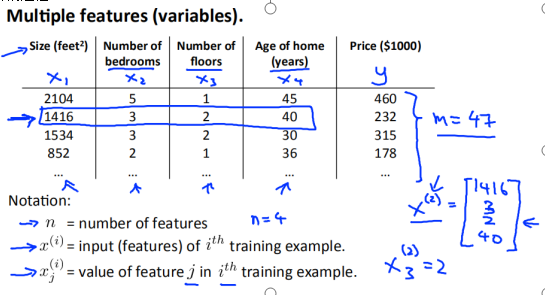如图所示输入特征为（x1,x2,x3,x4)

输出特征为y

样本总数为m

这时候我们假设拟合函数为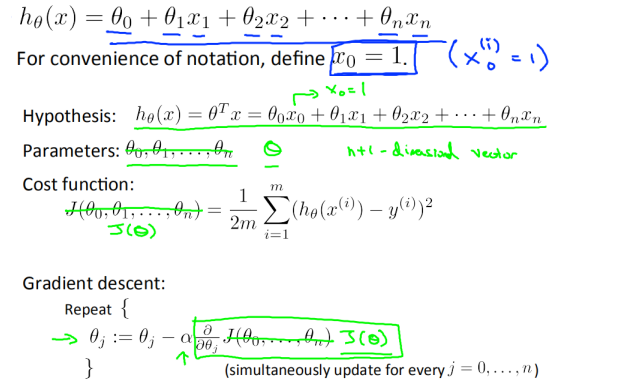那么我们要使代价函数最小时，参数的求解如图所示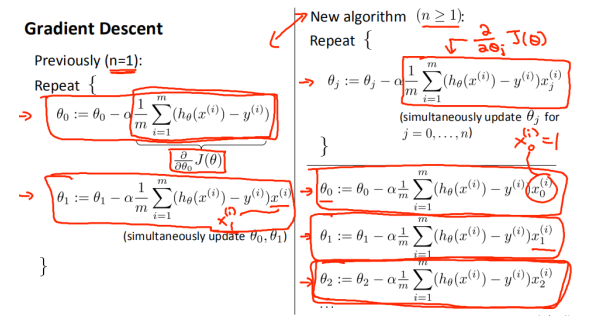为了便于理解代价函数在只有两个变量时，代价函数如图所示

X1 = size (0-2000)

X2 = bedroom (0-5)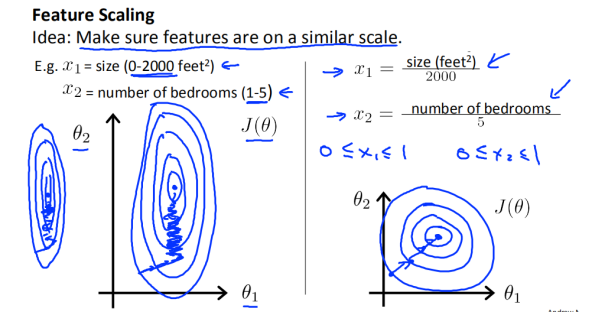通过观察左图我们发现x1与x2相差较大时，代价函数图像为瘦高形状，此时梯度下降到最优解时路线较为曲折，即回归难度较大，当参数较为相近时，如右图所示，梯度下降路线比较接近线性直线，即梯度下降较为容易。

所以我们需要将特征进行缩放

方法一 缩放到【0-1】 feature/ the total of number

方法二 缩放到【-1，1】 （feature - 均值）/ the total of number

当然如图 如果参数差距不大 比如在【-3，3】之间就没有必要去特征缩放了

在前面一节中，我们谈论了梯度下降时a对回归的影响，接下来我们具体讨论一下a的取值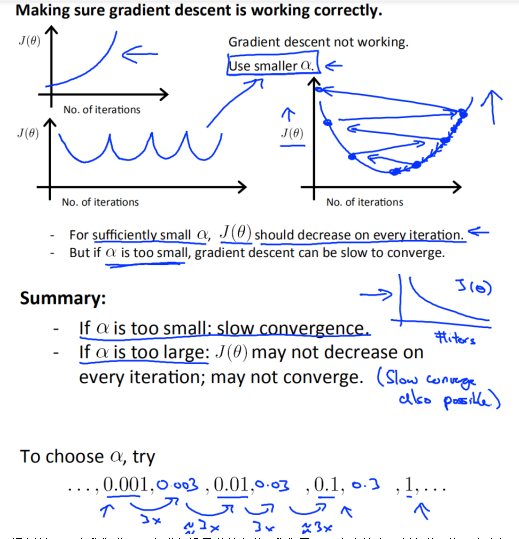通过前面观察我们发现要想求假设函数的参数，我们需要一步步的去更新参数，接下来介绍另外一中求解方式（通过数学推理的方法：正规方程 ，一步到位求出最优的参数）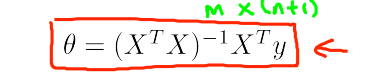我们观察一下这个公式，X为行向量，（XTX) 为n *n 的矩阵 ，要求解它的逆矩阵需要O(n3)复杂度，所以当特征较多时，我们不采用这种方法。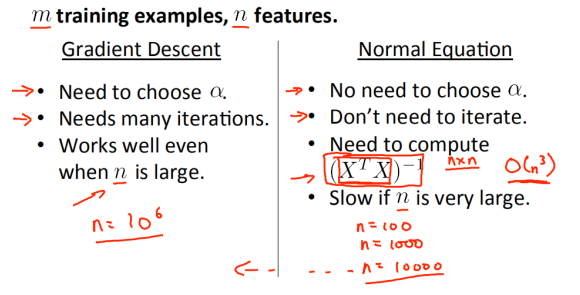展开全文机器学习
• 梯度下降法的基本思想非常简单，想象一下自己在一个盆地中，现在需要进入到盆地最底部，那么最简单的方式就是一直往下走，直到不能再向下走为止，此时就到达了盆地最底部。那么对于一个复杂的函数而言，找到一个合适...
符号说明
$\textbf{x},\textbf{x}_0,\textbf{x}_t,\triangle\textbf{x}$都是nx1的列向量。
$\textbf{x}_{t,i}$代表向量$\textbf{x}_t$的第i个元素，是个常量。
$f(\textbf{x})$为$\textbf{x}$的函数，是一个常量。

基本思想
梯度下降法的基本思想非常简单，想象一下自己在一个盆地中，现在需要进入到盆地最底部，那么最简单的方式就是一直往下走，直到不能再向下走为止，此时就到达了盆地最底部。那么对于一个复杂的函数而言，找到一个合适的公式去描述这一简单的思想，就可以求出函数的极小值位置了。

算法推导
高维函数带有佩亚诺余项的一阶泰勒展开式如下
$f(\textbf{x})=f(\textbf{x}_0)+\triangledown{f(\textbf{x}_0)}^T(\textbf{x}-\textbf{x}_0)+o(||\textbf{x}-\textbf{x}_0||)$
令$\textbf{x}=\textbf{x}_0+\triangle{\textbf{x}}$
$f(\textbf{x}_0+\triangle{\textbf{x}})-f(\textbf{x}_0)=\triangledown{f(\textbf{x}_0)}^T\triangle{}\textbf{x}+o(||\triangle{}\textbf{x}||)$
假设$\textbf{x}_0$处是极小值位置
那么下式成立
$\lim_{||\triangle{}\textbf{x}||\to 0}f(\textbf{x}_0+\triangle{\textbf{x}})-f(\textbf{x}_0)>0\tag{1.1}$
$\iff$
$\lim_{||\triangle{x}|| \to 0}\frac{\triangledown{f(\textbf{x}_0)}^T\triangle{}\textbf{x}}{||\triangle{\textbf{x}}||}+\lim_{\triangle{\textbf{x}}\to 0}\frac{o(||\triangle{\textbf{x}}||)}{||\triangle{\textbf{x}}||}>0\tag{1.2}$
$\iff$
$\lim_{||\triangle{x}|| \to 0}\frac{\triangledown{f(\textbf{x}_0)}^T\triangle{}\textbf{x}}{||\triangle{\textbf{x}}||}>0\tag{1.3}$
$\iff$
$\lim_{||\triangle{x}|| \to 0}\triangledown{f(\textbf{x}_0)}^T\triangle{}\textbf{x}>0\tag{1.4}$
可以得到如果两个向量$\triangledown{f{(\textbf{x}_0)}}$与$\triangle{\textbf{x}}$同向那么满足推导(4)，同样也满足公式(1)
$\lim_{||\triangle{\textbf{x}||\to0}}\triangle{\textbf{x}}=\alpha{\triangledown{f(\textbf{x}_0)}},\alpha>{0}$
令$\triangle{\textbf{x}}=\textbf{x}_{t}-\textbf{x}_{t+1}$，若要使$\triangledown{f(\textbf{x}_t)}$与其同向，只需要一个可收敛的递推式（可收敛很重要）
$\textbf{x}_{t}-\textbf{x}_{t+1}=\alpha{\triangledown{f(\textbf{x}_t)}},\alpha>{0}$
得到最终的梯度下降递推式如下
$\textbf{x}_{t+1}=\textbf{x}_{t}-\alpha{\triangledown{f(\textbf{x}_t)}},\alpha>{0},t=1,2,3,\cdots\tag{1.5}$
推导过程中假设条件为极小值，那么对于求极大值的情况常常在函数前面加一个负号，转换成求极小值的情况。
在应用中也会发现，有些存在极值的函数利用梯度下降无法求出极值，一般是$\alpha$取值或者函数本身的问题导致递推式无法收敛。从推导过程中可以看出，如果这个递推式不收敛，那么就不会满足推导(4)从而无法利用这个方法求极值。

算法改进
Polysk’s Classical Momentum算法
简称为CM算法，可以加速梯度下降法，递推公式如下
$\textbf{x}_{t+1}=\textbf{x}_t+\textbf{v}_{t+1}\tag{2.1}$
$\textbf{v}_{t+1}=u\textbf{v}_t-\alpha{\triangledown_{\textbf{x}_t}{f}},u\in{[0,1)}\tag{2.2}$
利用公式(2.2)对公式(2,1)展开，
$\textbf{v}_{t+1}=\textbf{u}^{t+1}\textbf{v}_0-\alpha{\textbf{u}^{t}}{\triangledown_{\textbf{x}_0}{f}}-\alpha{\textbf{u}^{t-1}}{\triangledown_{\textbf{x}_1}{f}}\cdots-\alpha{{\triangledown_{\textbf{x}_t}{f}}}\tag{2.3}$
$\textbf{x}_{t+1}=\textbf{x}_t+\textbf{v}_{t+1}\tag{2.4}$
一般而言，$\textbf{u}^{t+1}\textbf{v}_0$（一般为0向量）对$\textbf{v}_{t+1}$的方向影响几乎可以忽略，若该递推式是收敛的，那么该公式满足递推(1.4)，那么该递推式可以求出极值点，而且$\textbf{v}_{t+1}$变化量明显大于$-\alpha{{\triangledown_{\textbf{x}_t}{f}}}$所以当选取的初始迭代点$\textbf{x}_0$距离极值点较远时能够较快地达到收敛位置。但是缺点是会在即将到达收敛的时候在极值点附近来回震荡，所以也有可能导致更多的迭代步骤。左侧为梯度下降法的迭代示例，右侧为CM算法迭代步骤。与之前的分析一致。
Nesterov’s Accelerated Gradient算法
简称为NAG算法，也可以对对梯度下降法进行加速，其递推式如下
$\textbf{x}_{t+1}=\textbf{x}_t+\textbf{v}_{t+1}\tag{2.5}$
$\textbf{v}_{t+1}=u\textbf{v}_t-\alpha{\triangledown_{\textbf{x}_t+\textbf{v}_t}{f}},u\in{[0,1)}\tag{2.6}$
与CM算法相比，求梯度的位置不一样，CM算法是对当前的位置$\textbf{x}_t$的求梯度，NAG算法是对即将到达的下一个位置$\textbf{x}_t+\textbf{v}_t$求梯度，仅仅这一点的改变就可能会使其迭代步骤低于CM算法。
Adgard算法
迭代公式如下
$\textbf{x}_{t+1,i}=\textbf{x}_{t,i}-\frac{\alpha}{\sqrt{G_{t,i}+\epsilon}}\triangledown_{\textbf{x}_{t,i}}{f}\tag{2.7}$
$G_{t,i}=\sum_{i=0}^{i=t}\triangledown_{\textbf{x}_{t,i}^2}{f}\tag{2.8}$
随着迭代步骤t的增加，与梯度下降法中的$\alpha$相比，Adgard算法中的系数$\frac{\alpha}{\sqrt{G_{t,i}+\epsilon}}$越来越小，这种求解方式有效地减少了在极值点附近的震荡次数，但是如果选取的初始点距离极值点附近比较远，迭代次数将大幅上升。
RMSprop算法
该算法主要针对Adgard算法在极值点附近震荡过多，而提出的，迭代公式如下
$\textbf{x}_{t+1,i}=\textbf{x}_{t,i}-\frac{\alpha}{\sqrt{g_{t,i}+\epsilon}}\triangledown_{\textbf{x}_{t,i}}{f}\tag{2.9}$
$g_{t,i}=\gamma{G_{t-1,i}}+(1-\gamma){G_{t,i}}\tag{2.10}$
$G_{t,i}=\sum_{i=0}^{i=t}\triangledown_{\textbf{x}_{t,i}^2}{f}\tag{2.11}$
添加公式(2.10)，可以缓解在极值点附近的震荡次数。
Adam算法
前面我们提到CM算法与NAG算法减少迭代次数，但是在极值点附近常常出现过多的震荡，RMSprop算法与Adam算法可以有效降低这种震荡，但是开始的迭代次数比较多。所以有人就思考将两者结合起来，总体思想就是将CM算法作为分子，Adgard算法作为分母，期望达到在离极值点较远的时候分子不断增加从而减少初始迭代步骤，在离极值点较近的时候分母不断增加从而减少震荡的目的，由于公式过于臃肿，而且参数过多，导致本人不愿意敲打该公式，所以将原论文(Adam: A Method for Stochastic Optimization)中的伪代码粘贴到下面以表敬意。展开全文机器学习 算法
• 提前祝大家中秋节快乐！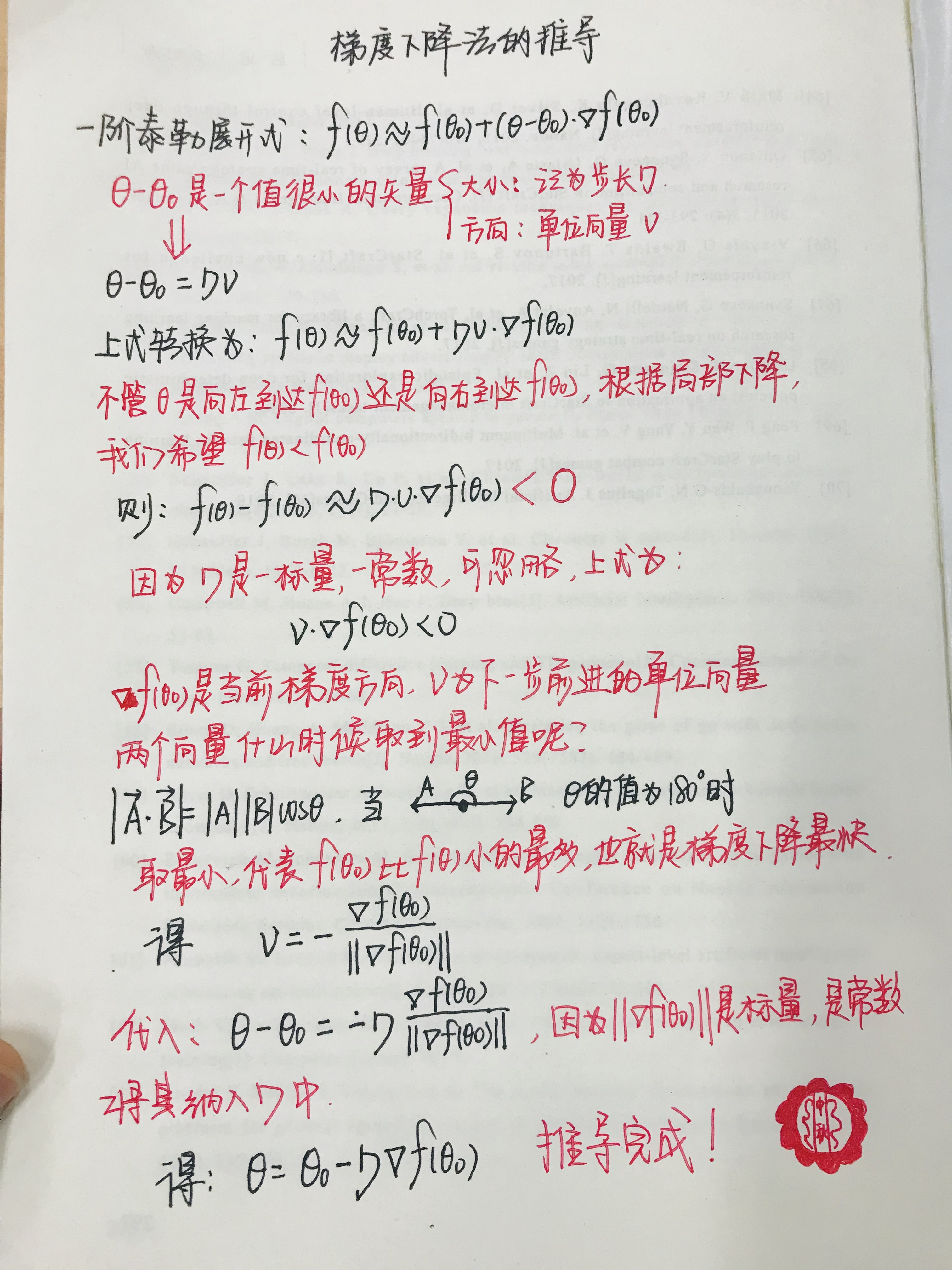提前祝大家中秋节快乐！

展开全文• 梯度下降方向更新 θ \theta θ ： θ j : = θ j − α ∂ J ( θ ⃗ ) ∂ θ j \theta_j := \theta_j-\alpha\frac{\partial J( \vec{\theta})}{\partial \theta_j} θ j ​ : = θ j ​ − α ∂ θ j ​ ∂ J...
• 由于梯度是上升最快的方向，而我们寻找的是下降最快的方向，故对于权值的更新规则： 其中η是一个正的常数，称为学习速率，决定了下降步长。是w(向量，符号打不出来)当前的权值向量，▽w(向量，符号打不...神经网络算法 机器学习
• 而本文讨论的姿态融合算法叫做梯度下降法，这部分代码可以参见Sebastian O.H. Madgwick在2010年4月发表的一篇论文（An efficient orientation filter for inertial andinertial/magneticsensor arrays），这篇论文...四元数姿态解算 四旋翼飞行器 四轴
• 梯度下降的内容还是比较重要的，希望早日入门机械学习，立帖为证。内容借鉴 沐雨金鳞 ，但是我觉得他的文章有些地方感觉比较模糊，因此以下的内容是在理解他的文章后，重新编排。若有内容上的错误，请各位大佬指教！机器学习 线性代数
• 转载自：原文 令w^=[wTb]T，对于最大化似然函数 ...注意是复合求导，其中sigmoid函数的导数为σ′(x)=σ(x)(1−σ(x))，因此计算w的第j个分量的梯度为 ∇wj===∑i=1My(i)1σ(w^x(i))σ(w^x(i))(1−σ(w^x(i)))
• 从别人的参考过来的推导过程梯度下降法实现对率回归数据集代码# -*- coding: utf-8 -*- from log import * import pandas as pd from pandas import * import matplotlib.pyplot as plt import numpy as np from ...机器学习
• # 简单线性回归（梯度下降法） # 0. 引入依赖 import numpy as np import matplotlib.pyplot as plt # 1. 导入数据（data.csv） points = np.genfromtxt('D:\学习资料\推荐系统\代码\练习代码\自己手打\data.csv'...算法 机器学习 python
• 梯度下降法 直观理解 当ω在曲线右半部分，导数>0，ω更新后会变小，向中间靠拢。反之，当ω在曲线左半部分，导数<0，ω更新后会变大，也向中间靠拢。 推导 方法1 泰勒展开，δ是变化量。当δ的方向...
• 梯度下降法是一个一阶最优化算法，通常也称为最陡下降法，要使用梯度下降法找到一个函数的局部极小值，必须向函数上当前点对应梯度的反方向的规定步长距离点进行迭代搜索。如果相反地向梯度正方向迭代进行搜索，则会...机器学习 数学
• 梯度下降法 梯度下降法是求解无约束最优化问题的一种最常用的方法，是一种迭代算法，每一步需要求解目标函数的梯度向量。 梯度的定义： 某一函数沿着某点处的方向导数可以以最快速度到达极大值，该方向导数我们...
• 没关系，接下来我将以通俗的语言来详细解释梯度下降算法公式的数学推导过程。下山问题假设我们位于黄山的某个山腰处，山势连绵不绝，不知道怎么下山。于是决定走一步算一步，也就是每次沿着当前位置最陡峭最易下山的...
• 这里就对梯度下降法做一个完整的总结。 　1. 梯度下降法的具体描述，请点击链接：刘建平Pinard梯度下降（Gradient Descent）小结。他已经将梯度下降的方方面面都讲得很详细了。 　2. 本文补充两点： 　①梯度下降...
• 摘要：梯度下降法是机器学习中用到比较多的一种求解方法，一般大家会通过形象化的图像来理解为什么梯度下降法...本文用数学推导的方式来证明梯度下降法有效，同时也介绍下牛顿法。 关键字：机器学习, 梯度下降, 牛顿法机器学习
• 文章目录前言公式推导总结 前言 什么是AI？ The theory and development of ...其实不用泰勒展开式也是可以简单方便的得出梯度下降法的结论的，在高数书本（同济）的微分的定义里就有讲到（我看的是同济7版第113页和
• 感知机的损失函数： L(w,b)=−∑xi∈Myi(w⋅xi+b)(1) L(w, b) = - \sum_{x_i \in M}y_i (w \cdot x_i + b) \tag {1} ...使用梯度下降法求出L(w,b)L(w,b)L(w,b)\$的偏导，使w,b向导数的负方向移动。 {∇wL(w,b)=−...
• 梯度下降是是无约束线性规划中求解最优问题最基本的方法，类似的还有牛顿，拟牛顿等。在解决问题的时候我们通常用来拟合f(x)，得到相关参数的值，然后进行预测。梯度在求解问题的时候总是选择当前位置的负梯度...线性回归
• 梯度下降法中，依然是通过加速度计的数据来与陀螺仪的数据进行融合，而不同点是：互补滤波法中，先求出载体坐标系b下对加速度做叉积求出误差，补偿给陀螺仪以校正误差；梯度下降法中，通过加速度计的数据求出一组...姿态解算 数学 数据
• 设hθ(x)=11+exp(−θTx)，且(x(i),y(i))为样本对，共有m个样本。 则极大似然函数有： l(θ)=log(∏i=1mhθ(xi)y(i)(1−hθ(x(i))1−y(i))=∑i=1m...梯度下降法求出似然函数最小值: ∂l(θ)∂θ=∑i=1m[y(i)hθ(xi...